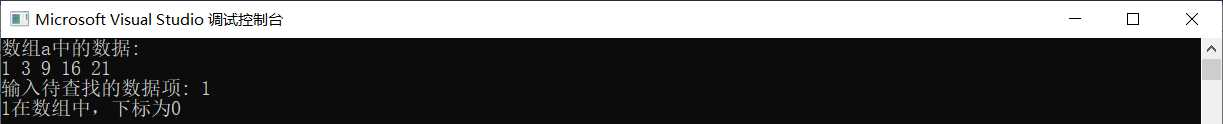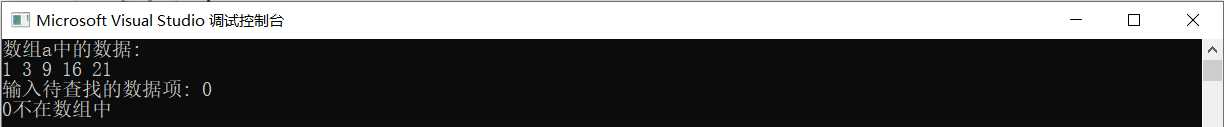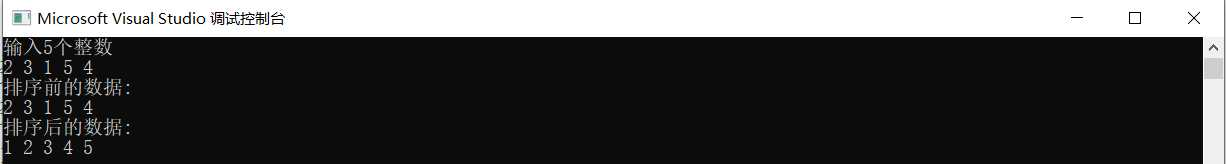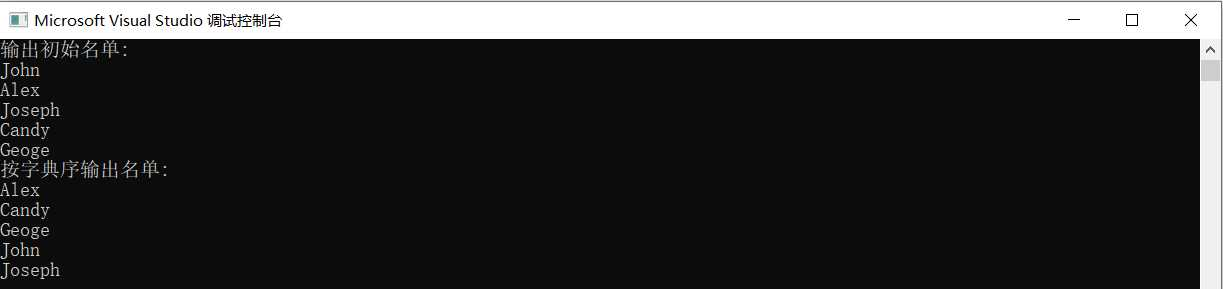# 数组和指针

Part 1

①利用数组

```// 练习：使用二分查找，在一组有序元素中查找数据项
//  形参是数组，实参是数组名
#include  <stdio.h>
const int N=5;
int binarySearch(int x[], int n, int item);
int main() {
int a[N]={1,3,9,16,21};
int i,index, key;

printf("数组a中的数据:\n");
for(i=0;i<N;i++)
printf("%d ",a[i]);
printf("\n");

printf("输入待查找的数据项: ");
scanf_s("%d", &key);

// 调用函数binarySearch()在数组a中查找指定数据项item,并返回查找结果给index
// 补足代码①
index = binarySearch(a,N,key);

if(index>=0)
printf("%d在数组中，下标为%d\n", key, index);
else
printf("%d不在数组中\n", key);

return 0;
}

//函数功能描述：
//使用二分查找算法在数组x中查找特定值item，数组x大小为n
// 如果找到，返回其下标
// 如果没找到，返回-1
int binarySearch(int x[], int n, int item) {
int low, high, mid;

low = 0;
high = n-1;

while(low <= high) {
mid = (low+high)/2;

if (item == x[mid])
return mid;
else if(/*补足代码②*/item < x[mid])
high = mid - 1;
else
low = mid + 1;
}

return -1;
}```

②利用指针

```// 练习：使用二分查找，在一组有序元素中查找数据项
//  形参是指针变量，实参是数组名
#include  <stdio.h>
const int N=5;
int binarySearch(int *x, int n, int item);
int main() {
int a[N]={1,3,9,16,21};
int i,index, key;

printf("数组a中的数据:\n");
for(i=0;i<N;i++)
printf("%d ",a[i]);
printf("\n");

printf("输入待查找的数据项: ");
scanf_s("%d", &key);

// 调用函数binarySearch()在数组a中查找指定数据项item,并返回查找结果
// 补足代码①
index = binarySearch(a, N, key);

if(index>=0)
printf("%d在数组中，下标为%d\n", key, index);
else
printf("%d不在数组中\n", key);

return 0;
}

//函数功能描述：
//使用二分查找算法在x指向的数据项开始的n个数据中，查找item
// 如果找到，返回其位置
// 如果没找到，返回-1
int binarySearch(int *x, int n, int item) {
int low, high, mid;

low = 0;
high = n-1;

while(low <= high) {
mid = (low+high)/2;

if (item == *(x+mid))
return mid;
else if(/*补足代码②*/item < *(x + mid))
high = mid - 1;
else
low = mid + 1;
}

return -1;
}```Part 2

①对一组数据由小到大排序

1. 第1遍，从a到a[n-1]中，选出最小的元素，将它与a交换。
2. 第2遍，从a到a[n-1]中，选出最小的元素，将它与a交换。
3. ...
4. 第n-1遍，从剩余2个数据项中，选出最小的元素与a[n-2]交换 至此，排序过程结束。
```// 示例: 使用选择法排序对一组整数由小到大排序
#include <stdio.h>
const int N=5;
void selectSort(int [], int);   // 函数声明（函数声明中可以省略变量名、数组名）
void input(int [], int);
void output(int [], int);
int main() {
int a[N];

printf("输入%d个整数\n", N);
input(a, N);

printf("排序前的数据:\n");
output(a,N);

selectSort(a,N); // 调用selectSort()对数组a中的N个元素排序

printf("排序后的数据:\n");
output(a, N);

return 0;
}

// 函数定义
// 函数功能描述：输入n个整数到数组a中
void input(int a[], int n) {
int i;
for(i=0; i<n; i++)
scanf_s("%d", &a[i]);
}

// 函数定义
// 函数功能描述：输出数组a中的n个整数
void output(int a[], int n) {
int i;
for(i=0; i<n; i++)
printf("%d ", a[i]);
printf("\n");
}

// 函数定义
// 函数功能描述：使用选择法对数组a中的n个整数由小到大排序
void selectSort(int a[], int n) {
int i, j, k, temp;

for(i=0; i<n-1; i++) {
k = i;  // k用于记录当前最小元素的下标

for(j=i+1; j<n; j++)
if (a[j] < a[k])
k = j;   // 如果a[j]比当前最小元素还要小，就更新k，确保它总是存放最小元素的下标

if(k != i) {  // 找到最小元素后，交换a[i]和a[k]
temp = a[i];
a[i] = a[k];
a[k] = temp;
}
}```②对一字符串按字典序排序

```// 练习：使用选择法对字符串按字典序排序
#include <stdio.h>
#include <string.h>
void selectSort(char str[], int n ); // 函数声明，形参str是二维数组名
int main() {
char name[] = {"John", "Alex", "Joseph", "Candy", "Geoge"};
int i;

printf("输出初始名单:\n");
for(i=0; i<5; i++)
printf("%s\n", name[i]);

selectSort(name, 5);  // 调用选择法对name数组中的字符串排序

printf("按字典序输出名单:\n");
for(i=0; i<5; i++)
printf("%s\n", name[i]);

return 0;
}

// 函数定义
// 函数功能描述：使用选择法对二维数组str中的n个字符串按字典序排序
void selectSort(char str[], int n) {
// 补足代码
int i, j, k;
char temp;

for (i = 0; i < n-1; i++) {
k = i;

for (j = i+1; j<n; j++)
if (strcmp(str[j], str[k]) < 0)
k = j;

if (k != i) {
strcpy_s(temp, str[i]);
strcpy_s(str[i], str[k]);
strcpy_s(str[k], temp);
}
}
}```Part 3

编写子函数 delPre?xStar()，删除字符串中所有前导*删除，中间的和后面的*不删除。

即删除后，字符串的内容应当是 A*BC*DEF*G*******

```// 函数定义
// 函数功能描述
// 删除字符数组s中前导*
void delPrefixStar(char s[]) {
char *target, *source;

// 从字符串开始找到不是*的位置
source = s;
while(*source == ‘*‘)
source++;

// 从这个位置开始将余下的字符前移
target = s;
while( *target++ = *source++);
}```

编写子函数 delStarButPre?x()，除了前导*之外，删除其它*

即删除后，字符串的内容应当是****ABCDEFG

```// 函数定义
// 函数功能描述
// 删除字符数组s中除了前导*以外的所有*（即删除字符串中间和末尾出现的*）
void delStarButPrefix(char s[]) {
int i=0;              // i用于记录字符在字符数组s中的下标
char *p = s;

// 跳过前导*,i记录字符在字符数组s中的下标，p记录首个非*字符的位置
while(*p && *p == ‘*‘) {
p++;
i++;
}

// 从p指向的字符开始,把遇到的*删除
while(*p) {
if(*p != ‘*‘) {
s[i] = *p;
i++;
}
p++;
}

s[i] = ‘\0‘;   // 思考：这一步这样写的原因
}```

’\0’是判定字符数组结束的标识，表示这串字符到结尾了

编写子函数 delMiddleStar()，除了前导*和尾部*之外，删除中间出现的所有*

即删除后，字符串内容应当是 ****ABCDEFG*******

```// 函数定义
// 函数功能描述
// 对字符数组s中存放的字符串，删除中间出现的*
void delMiddleStar(char s[]) {
int i=0;
char *tail, *head, *p;

// 找到末尾第一个非*字符的位置
tail = s;
while(*tail)
tail++;

tail--;

while(*tail == ‘*‘)
tail--;

// 找到开头第一个非*字符的位置

// 把中间出现的*去掉
p = s;
while(p<=head) {  // 思考这里条件表达式为什么这样写，这个循环的功能？
s[i] = *p;
p++;
i++;
}

while(p<tail) {
if(*p != ‘*‘) {
s[i] = *p;
i++;
}

p++;
}

while(*p) {
s[i] = *p;
i++;
p++;
}

s[i] = ‘\0‘; // 思考这里为什么要这样做
}```

1. 将s赋给了p
4. 第三个循环while(*p)便是将tail之后的字符赋给s
5. 关于‘\0’同上

Part 4

1、字符串比较函数

（1）字符串1=字符串2，返回0

（2）字符串1>字符串2，返回一个正整数

（3）字符串1<字符串2，返回一个负整数。

2、字符串复制函数

str1,str2是两个字符串数组的数组名

3、指针和数组要掌握的真的挺多的啊，而且运用特别灵活，这个部分得好好学学（￣︶￣）↗

(0)
(0)

© 2014 bubuko.com 版权所有 鲁ICP备09046678号-4